Specific intensity of radiation fieldsis defined by the radiation energy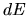transferred by photons with wavelength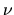through a unit surface placed at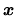whose normal is directed to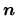per unit time per unit wavelength, and per unit steradian. Average intensity of radiation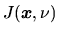is defined as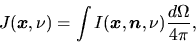(2.121)

where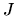is obtained by averaging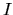for the solid angle. This is related to the energy density of radiation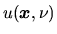as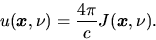(2.122)

If the radiation is absorbed in the displacement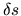as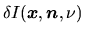,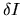must be proportional toandas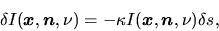(2.123)

where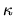is a coefficient and called volume absorption coefficient. The dimension ofis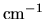. We can rewrite the above to the differential equation as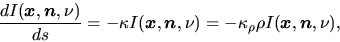(2.124)

where we used mass absorption coefficient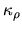which represents the absorption per mass. Equation (2.123) is reduced to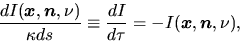(2.125)

where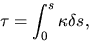(2.126)

is called the optical depth. This means that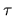is a measure for absorption as the intensity decreases at a factor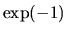from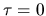to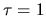.

If the ray runscrossing a volume whose volume emissivity equal to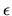the intensity increases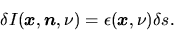(2.127)

The volume emissivity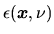is the energy emitted by a unit volume at a positionper unit time per unit solid angle per unit wavelength. From equations (2.123) and (2.127), the radiation transfer is written as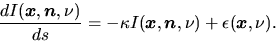(2.128)

Using the optical denpth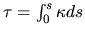, this gives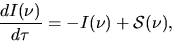(2.129)

where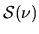is called the source function and is defined as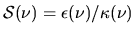. Assuming the specific intensity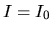at the point of, that at the pointon the same ray is given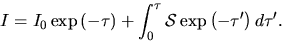(2.130)

In the case of constant source term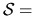const it reduces to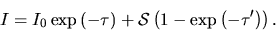(2.131)

Equation (2.130) gives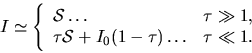(2.132)

This indicates that if we see an optically thick cloud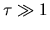the specific intensity reaches us represents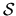, while if we see an transparent cloud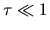,represents that of background.

Problem
Show that equations (2.130) and (2.130) are solutions of equation (2.129).